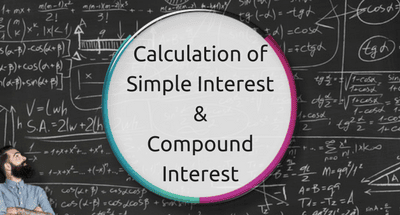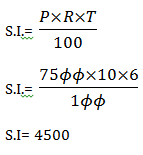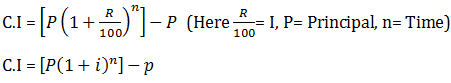# Calculation of Simple Interest and Compound Interest for JAIIB## Simple Interest Definition

### Principals (P):

The original Sum of Money Loaned/deposited.

### Interest (I):

The amount of money that you pay to borrow money or the amount of money that you earn on a deposit

### Time (T):

The duration for which the money is borrowed/deposited.

### Rate of Interest (R):

the percent of interest that you pay for money borrowed, or earn for money deposited.

## Formula for Calculating S.IWhere
P= Principal
R- Rate of Interest (in %)
T = Time period.

### Examples:

#### Question. What is the S.I on 7500/- at the rate of 10% per annum for 6 years

Solution:Solution:

## Compound Interest

### Definition

Internal calculation on initial principal and also on the accumulated interest of previous periods of deposit or loan

### Formula:

Formula for calculation of compound interest#### For Example:

A took three years loan of Rs. 10000 at an interest rate of 5% that compound annually. What would be the compound interest?### Elaboration

 Year Opening Balance(P) Interest (I) 5% Closing Balance (P+I) 1. 10000 500 10,500.00 2. 10500 525 11025 3. 11025 551 11576

#### Compound Interest Payment can be made

• Monthly = 12
• Quarterly = 4
• Semi- annually= 2
• Annually = 1.

(It means I should be divided by no. of time it is compounded)

#### For Example:

Ram invested Rs. 2000 for 2 years at the rate of 5% that is compounded annually. What will be C.I?Taking the same example compounded MonthlyJAIIB Classes by Ramandeep Singh - Get details here